## Access NCERT Solutions for Class 6 Maths Chapter 14: Practical Geometry Exercise 14.1

### Access NCERT Solutions for Class 6 Maths Chapter 14: Practical Geometry Exercise 14.1

1. Draw a circle of radius 3.2 cm.

Solutions:

The required circle may be drawn as follows:

Step 1: For the required radius 3.2 cm, first open the compasses.

Step 2: For the centre of a circle, mark a point ‘O’.

Step 3: Place a pointer of compasses on ‘O’.

Step 4: Now, turn the compasses slowly to draw the required circle.2. With the same centre O, draw two circles of radii 4 cm and 2.5 cm.

Solutions:

The required circle may be drawn as follows:

Step 1: For the required radius 4 cm, first open the compasses

Step 2: For the centre of a circle, mark a point ‘O’

Step 3: Place a pointer of compasses on ‘O’.

Step 4: Turn the compasses slowly to draw the circle.

Step 5: Next, open the compasses for 2.5 cm.

Step 6: Again place a pointer of compasses on ‘O’ and turn the compasses slowly to draw the circle.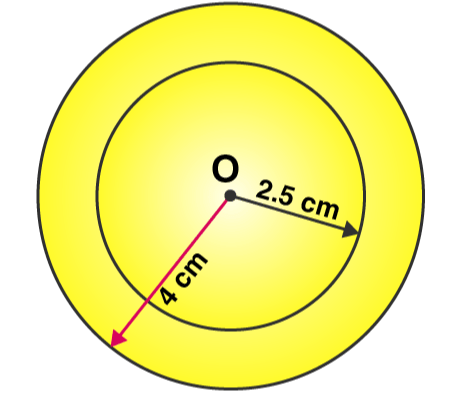3. Draw a circle and any two of its diameters. If you join the ends of these diameters, what is the figure obtained? What figure is obtained if the diameters are perpendicular to each other? How do you check your answer?

Solutions:

We may draw a circle having its centre ‘O’, also of any convenient radius. Let AB and CD be the two diameters of this circle. A quadrilateral is formed when we join the ends of these diameters.We know that the diameter of a circle are equal in length, hence quadrilateral formed will be having its diagonals of equal length.

Also, OA = OB = OC = OD = radius r and if a quadrilateral has its diagonals of same length bisecting each other. It will be a rectangle.

Let DE and FG be the diameters of the circle such that both are perpendicular to each other. Now we can find that a quadrilateral is formed by joining the ends of these diameters.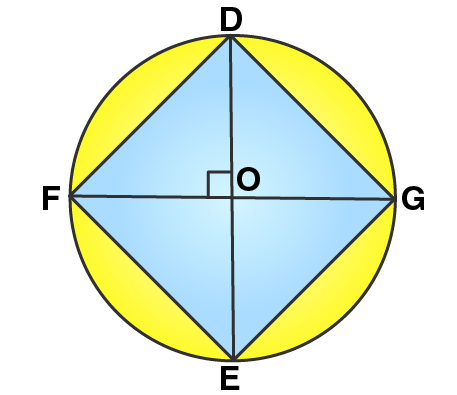We may find that OD = OE = OF = OG = radius r.

In this quadrilateral DFEG, diagonals are equal and perpendicular to each other. Also they are bisecting each other, hence it will be a square.

To check our answers we may measure the length of sides of quadrilateral formed.

4. Draw any circle and mark points A, B and C such that

(a) A is on the circle.

(b) B is in the interior of the circle.

(c) C is in the exterior of the circle.

Solutions:

We may draw a circle and three required points A, B, C as follows:5. Let A, B be the centres of two circles of equal radii; draw them so that each one of them passes through the centre of the other. Let them intersect at C and D. Examine whether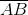and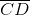are at right angles.

Solutions:

Let us draw two circles having with same radius which are passing through the centre of the other circle.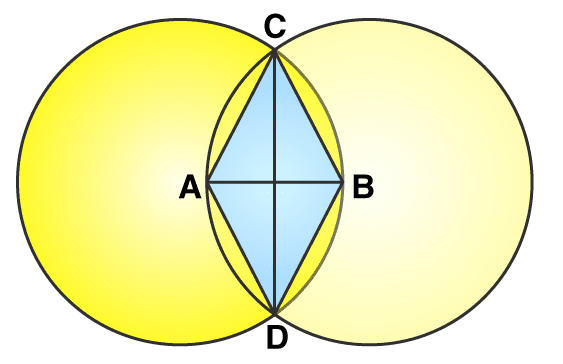Here points A and B are the centres of these circles and these circles are intersecting each other at points C and D respectively.

Hence quadrilateral ADBC is a rhombus and in rhombus diagonals bisect each other at 900. Henceandare at right angles.